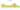# Lek

## Albanian Lek

Albania's national currency is the lek. It used to be divisible into 100 qintars, however there are no longer any qintar coins being produced.

#### how has the value of the currency changed in the last year?## USD 1 = ALL 99.444

How much is10 US dollars worth inAlbanian lekë?
At the current exchange rate, 10 US dollars is worth 994.44 Albanian lekë
How much is50 US dollars worth inAlbanian lekë?
At the current exchange rate, 50 US dollars is worth 4,972.21 Albanian lekë
How much is100 US dollars worth inAlbanian lekë?
At the current exchange rate, 100 US dollars is worth 9,944.42 Albanian lekë
How much is500 US dollars worth inAlbanian lekë?
At the current exchange rate, 500 US dollars is worth 49,722.09 Albanian lekë
How much is2,000 US dollars worth inAlbanian lekë?
At the current exchange rate, 2,000 US dollars is worth 198,888.34 Albanian lekë
How much is10 US dollars worth inAlbanian lekë?
At the current exchange rate, 10 US dollars is worth 994.44 Albanian lekë
How much is50 US dollars worth inAlbanian lekë?
At the current exchange rate, 50 US dollars is worth 4,972.21 Albanian lekë
How much is100 US dollars worth inAlbanian lekë?
At the current exchange rate, 100 US dollars is worth 9,944.42 Albanian lekë
How much is500 US dollars worth inAlbanian lekë?
At the current exchange rate, 500 US dollars is worth 49,722.09 Albanian lekë
How much is2,000 US dollars worth inAlbanian lekë?
At the current exchange rate, 2,000 US dollars is worth 198,888.34 Albanian lekë